# How to Determine the Limits of Functions

Lesson Transcript
Instructor
Zach Pino
Expert Contributor
Simona Hodis

Simona received her PhD in Applied Mathematics in 2010 and is a college professor teaching undergraduate mathematics courses.

To find the limit of a continuous function, use substitution. Learn more about this rule and more about the properties of limits and how to determine the limits of functions using examples. Updated: 08/09/2021

## Properties of Limits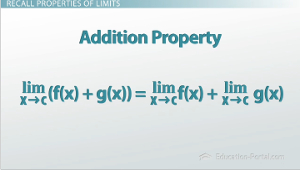So let's talk for a minute about calculating limits. Let's look at the function f(x) = (x - 3)sin(x) + 10. Let's find the limit of this function as x approaches 3.7. To do this, let's first recall properties of limits. We have an addition property that might be useful here. This says that the limit as x approaches C of some sum of two functions is equal to the limit of each one of those functions taken separately and added together. This is our 'divide and conquer' property. We have another 'divide and conquer' property when looking at products. This says that if you want to take the limit of two functions that are multiplied together, you can take the limit of each one of those functions separately and multiply the answer together. Again, this is 'divide and conquer.'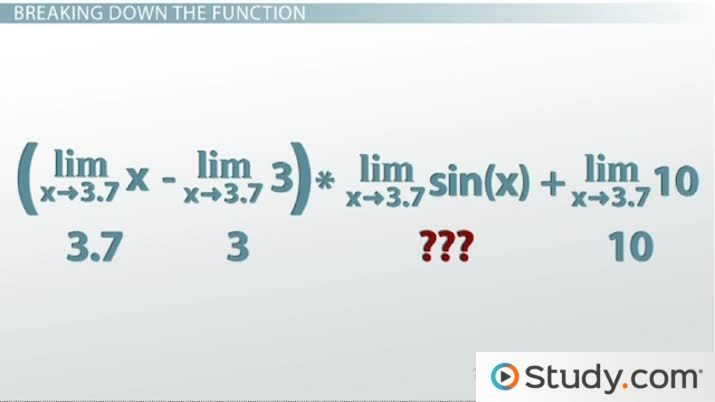An error occurred trying to load this video.

Try refreshing the page, or contact customer support.

Coming up next: Understanding the Properties of Limits

### You're on a roll. Keep up the good work!

Replay
Your next lesson will play in 10 seconds
• 0:06 Properties of Limits
• 1:00 Breaking Down the Function
• 3:16 Continuous Functions
• 4:48 Lesson Summary
Save Save

Want to watch this again later?

Timeline
Autoplay
Autoplay
Speed Speed

## Breaking Down the Function

If we go back to our function, f(x) = (x - 3)sin(x) + 10, and we want to find the limit as x goes to 3.7 of this function, we're going to look at these pieces individually. We're going to use the product rule to separate x - 3 from sin(x), we're going to use the addition rule to look at that + 10, and we're going to use the subtraction rule, which is just like the addition rule, to separate the x - 3 into x and 3. Now I'm pretty confident that if I graph out x and 3, I can show that as x goes to 3.7, x will also go to 3.7. As x goes to 3.7, 3 will go to 3. I'm also pretty confident that as x goes to 3.7, 10 will stay at 10. So I can plug in all these numbers except for this sin(x). So what do we do about that?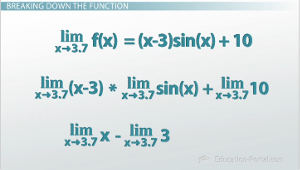To unlock this lesson you must be a Study.com Member.

## Limits of Functions - Solving Indeterminate Cases

### Review Key Terms

To evaluate a limit of x approaching a, where a is a finite number, we first substitute the value x = a in the function and, if we obtain a finite value, the limit is that finite value.

If the limit is an indeterminate case like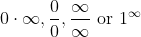then we have different techniques to eliminate the indeterminate case and evaluate the limit.

Some of the techniques are:

1. L'Hospital rule when we have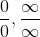and the limit is equal to the ratio of the derivative functions of the numerator and denominator functions.

2. Rationalizing a fraction that involves a sum of radicals

3. Factoring out and simplifying fractions.

4. Using logarithmic rules when the indeterminate case is {eq}1^\infty {/eq} and write the function as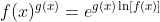5. Analyzing the end-behavior of functions, when we have limits at infinity.

### Applications

For each of the following limits, state the indeterminate case, if any, and the technique used to eliminate the indeterminate case and evaluate the limit.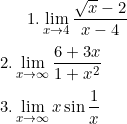### Solutions

1. The indeterminate case is 0/0 and we will rationalize the fraction with the conjugate of the numerator.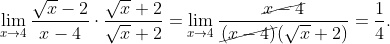2. The indeterminate case is infinity/infinity and we will factor out x and simplify.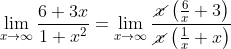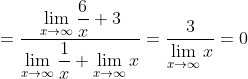3. The indeterminate case is and we solve it with L'Hospital, after we rewrite it as: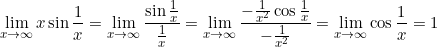### Register to view this lesson

Are you a student or a teacher?